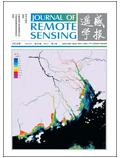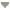出版日期: 2016-11-25点击次数：下载次数：DOI: 10.11834/jrs.201660602016 | Volumn20 | Number 6 上一篇|耿君1,2,3 , 田庆久1,2,3 , 涂丽丽1,2,3 , 范渭亮4 , 王晓菲1,2,3
1. 南京大学 国际地球系统科学研究所，江苏 南京 210023
2. 江苏省地理信息技术重点实验室，江苏 南京 210023
3. 江苏省地理信息资源开发与利用协同创新中心，江苏 南京 210023
4. 浙江农林大学 环境与资源学院，浙江 临安 311331
 收稿日期: 2016-03-03; 优先数字出版日期: 2016-11-25 基金项目: 国家科技重大专项项目(编号：03-Y20A04-9001-15/16, 30-Y20A29-9003-15/17) 第一作者简介: 耿君(1988—)，男，博士研究生，研究方向为植被定量遥感研究。E-mail： gaoyugengjun@163.com 通讯作者简介: 田庆久(1964—)，男，教授，研究方向为高光谱遥感、定量遥感研究。E-mail： tianqj@nju.edu.cn 中图分类号: TP79 文献标识码: A

# 关键词

Influences of crown size on the estimation of gap fraction and clumping indexGENG Jun1,2,3 , TIAN Qingjiu1,2,3 , TU Lili1,2,3 , FAN Weiliang4 , WANG Xiaofei1,2,3
1.International Institute for Earth System Science, Nanjing University, Nanjing 210023, China
2.Jiangsu Provincial Key Laboratory of Geographic Information Science and Technology, Nanjing 210023, China
3.Jiangsu Provincial Collaborative Innovation Center of Geographic Information Resources Development and Utilization, Nanjing 210023, China
4.College of Environmental and Resource Sciences, Zhejiang A & F University, Lin'an 311331, China

# Abstract

Gap fraction and foliage clumping index play key roles in plant light interception; therefore, they have strong influences on plant growth and canopy radiative transfer processes. Leaves always aggregate in crowns, which are important objects innumerous geometric-optical models of forests. Researchers have mainly focused on the influences of crown shape (e.g., cylinder, cone, ellipsoid, and cone + cylinder) on gap fraction and foliage clumping index. However, size is also an important characteristic of tree crowns. Crown sizes result from the interactions between plants and environments during the long-term evolution process of the plants. In fact, crown size characteristics have more obvious geographical spatial features than crown shape. Therefore, crown size should be given considerable attention when studying plant light interception and canopy radiative transfer processes in the fields of phytogeography, remote sensing and global change. The main objective of this study is to exhibit how crown size characteristics influence the gap fraction and foliage clumping index of forest canopies. First, a simple and general distance factor, which is defined as the relative allowable shortest distance between centers of any two crowns divided by the mean diameter of tree crowns, is proposed to quantitatively describe the degree of repulsion effect among trees in forest canopies. Second, the Poisson distribution model of trees is completely replaced by the hypergeometric model, which is more suitable for quantitatively describing the repulsion effect and spatial relationship among trees in forest stands. Finally, seven sizes of ellipsoids (from prolate ellipsoids to oblate ellipsoids) are selected for the tree crowns, and the influences of crown size on gap fraction and foliage clumping index are exhibited by means of fixing the radius and the volume of the tree crowns, respectively. Results show that the following: (1) the influences of crown size characteristics are significantly greater than the effects of crown shape on gap fraction and foliage clumping index, regardless if the radius or volume of tree crowns are fixed. (2) From prolate ellipsoids to oblate ellipsoids, crown size characteristics show significant and regular effects on both gap fraction and foliage clumping index. In extreme cases, when θ=60°, the canopy gap fraction when ellipsoid height ( H b) is 16 m is 92.8% lower than that when H b=0.25 m, and the foliage clumping index when H b=16 m is 261.8% greater than that when H b=0.25 m when the crown radius is fixed. At θ=0°, the canopy gap fraction when H b=16 m is 414.1% greater than that when H b=0.25 m, and the foliage clumping index when H b=0.25 m is 11397.2% greater than that when H b=16 m when the volume of crown is fixed. The larger the projected area of the crown in the observed direction, the lower value of the gap fraction and the higher value of the foliage clumping index, indicating that leaves tend to distribute randomly in forest canopies.

# Key words

crown size , gap fraction , clumping index , hypergeometric model , crown shape

# 1 引 言

 $P(\theta ) = \exp \left[ { - k*L/\cos \theta } \right]$ (1)

 $P(\theta ) = \exp \left[ { - k*L*\varOmega /\cos \theta } \right]$ (2)

# 2 理论与方法

## 2.1 林分内树冠的分布模式

Fig. 1 Schematic of the exclusion effects among trees in a forest stand (Solid circles represent crowns; green dash lines represent the exclusion distances which are the products of RASD and the mean diameter of the crowns; red zones mean pseudo reject areas and need to be removed when calculating GF and CI. Dash circles are the“crusts”of trees)

## 2.2 冠层间隙率

 $\frac{{S - {t_{\rm{c}}}}}{S} \! * \! \frac{{S - 2 {t_{\rm{c}}}}}{{S - {t_{\rm{c}}}}}*\frac{{S - 3 {t_{\rm{c}}}}}{{S - 2 {t_{\rm{c}}}}} \! * \! \ldots \! * \! \frac{{S - {N_{\rm{c}}}{t_{\rm{c}}}}}{{S - ({N_{\rm{c}}} - 1{\rm{)}}{t_{\rm{c}}}}} = \frac{{S - {N_{\rm{c}}}{t_{\rm{c}}}}}{S}$ (3)

 \begin{aligned} & {P_{{\rm{Ht}}}}(\theta ) = \\ & \prod\limits_{j = 1}^{{N_{\rm{c}}}} {\Bigg[1 - \frac{{{t_{\rm{c}}}(\theta )*(1 - {P_{\rm{c}}}(\theta ))}}{{S*\cos (\theta ) - {S_{{\rm{rej\_c}}}}(\theta )*(1 - {P_{\rm{c}}}(\theta ))*(j - 1)}}\Bigg]} \end{aligned} (4)

 \begin{aligned} & {S_{{\rm{rej\_c}}}}(\theta ) = \\ & \left\{ \begin{array}{l} {\!\!\! S_{{\rm{crust\_c}}}}(\theta )\;\;\;\;\;\;\;\;\;\;\;\;\;\;\;\;\;\;{R_{{\rm{ASD}}}} \leqslant 1\\ {\!\!\! S_{{\rm{crust\_c}}}}(\theta ) - {S_{{\rm{pse\_c}}}}(\theta )\;\;\;\;{R_{{\rm{ASD}}}} > 1;\;{R_{{\rm{ASD}}}}r\rm cos(\theta ) \leqslant {t_h}(\theta ) \\ {\!\!\! t_{\rm{c}}}(\theta )\;\;\;\;\;\;\;\;\;\;\;\;\;\;\;\;\;\;\;\;\;\;\;{R_{{\rm{ASD}}}} > 1;\;{R_{{\rm{ASD}}}}r\rm cos(\theta ) > {t_h}(\theta ) \end{array} \right. \end{aligned} (5)

 ${t_{\rm{c}}}(\theta ) = \left\{ \begin{array}{l} r*{\rm{\pi }}* \displaystyle \frac{{\sqrt {{k_{\rm{s}}}^2{r^2} + {{({H_{\rm{b}}}/2)}^2}} }}{{\sqrt {{k_{\rm{s}}}^2 + 1} }}\;\;\;\;\theta \ne 0^\circ\\ {r^2}*{\rm{\pi }}\;\;\;\;\;\;\;\;\;\;\;\;\;\;\;\;\;\;\;\;\;\;\;\;\;\;\;\;\;\;\;\;\theta = 0^\circ \end{array} \right.$ (6)

 ${P_{\rm{c}}}(\theta ) = \exp \left[ { - G(\theta )*{L_0}*{\varOmega _{\rm{c}}}/{\gamma _{\rm{e}}}} \right]$ (7)

 ${L_0} = {s_{_0}}*L*S/(V*{N_{\rm{c}}})$ (8)

 ${{P}_{\text{c}}}(\theta )=\exp \left[ -G(\theta )*L*S*09000{{\Omega }_{c}}/({{N}_{\text{c}}}*{{t}_{\text{c}}}(\theta )*{{\gamma }_{\text{e}}}) \right]$ (9)

## 2.3 冠层叶片聚集度指数

 $\varOmega (\theta ) = \frac{{\log ({P_{{\rm{Ht}}}}(\theta ))}}{{\log \left( {{P_{{\rm{Poisson}}}}(\theta )} \right)}}$ (10)

 $\varOmega (\theta ) = \frac{{{L_{\rm{e}}}}}{L}$ (11)

 $Le = - 2\int_0^{\pi /2} {\ln \left[ {P(\theta )} \right]\cos \theta \sin \theta {\rm{d}}\theta }$ (12)

# 3 结果与讨论

## 3.1 相同树冠半径下，不同树冠高度对冠层间隙率和叶片聚集度指数的影响

Table 1 Canopy structural parameters

 参数 值 林分面积/m 2 100×100 树冠数目 2000 叶面积指数 4 树干高度/m 0.5 树冠半径/m 1 树冠高度/m 0.25; 0.5; 1; 2; 4; 8; 16 G( θ) 0.5 Ω c 1 γ e 1 R ASD 1

Fig. 2 Comparison of gap fraction of canopies with same r but different H b

Fig. 3 Comparison of clumping index of canopies with same r but different H b

Fig. 4 Comparison of expectation of clumping index of canopies in hemisphere space with same r but different H b

## 3.2 相同树冠体积下，不同树冠尺寸对冠层间隙率和叶片聚集度指数的影响

3.1节中揭示了不同树冠尺寸下的冠层间隙率和叶片聚集度指数因树冠体积的不同表现出有规律的变化。本节通过固定单个树冠体积，同时调节树冠高度和树冠半径，讨论不同树冠尺寸对冠层间隙率和聚集度指数的影响。为防止当 R ASD=1时“矮胖型”树冠溢出林分边界，设定林分内树冠株数为360；树冠尺寸参数如 表2所示，其他参数参照 表1

Table 2 Crown sizes with same V

 树冠半径/m 树冠高度/m 2.83 0.25 2 0.5 1.41 1 1 2 0.71 4 0.5 8 0.35 16

Fig. 5 Comparison of clumping index of canopies with same V but different crown size

Fig. 6 Comparison of clumping index of canopies with same V but different crown size

Fig. 7 Comparison of expectations of clumping index of canopies in hemisphere space with same V but different crown size

# 4 结 论

(1) 揭示了树冠尺寸特征对冠层间隙率和叶片聚集度指数的影响比树冠形状特征对间隙率和叶片聚集度指数的影响更为明显，在大范围研究中更值得关注。

(2) 相同树冠半径下，不同树冠高度对冠层间隙率和叶片聚集度的影响显著，且具有明显的规律性：树冠越高对应的冠层间隙率越小，叶片聚集度越大，冠层内叶片越趋于泊松分布。例如，当 θ=60°， H b=16 m树冠下的冠层间隙率比 H b=0.25 m下的值低92.8%；当 θ=60°， H b=16 m树冠下的叶片聚集度比 H b=0.25 m下的值高261.8%； H b=16 m下的叶片聚集度指数期望值高于 H b=0.25 m下的值约250.8%。

(3) 相同树冠体积下，不同树冠尺寸对冠层间隙率和叶片聚集度的影响非常显著，且具有非常明显的规律性。球体树冠的叶片聚集度最小；树冠尺寸与球体的差异越大，叶片聚集度越大。例如， θ=0°时， H b=16 m下的冠层间隙率高于 H b=0.25 m下的值414.1%； H b=0.25 m下的叶片聚集度高于 H b=16 m下的值11397.2%； H b=16 m下的叶片聚集度期望值高于 H b=2 m下的值677.3%。

• Chen J M, Black T A.1992.Defining leaf area index for non-flat leaves. Plant, Cell & Environment, 15 : 421–429. DOI: 10.1111/j.1365-3040.1992.tb00992.x.
• Chen J M, Cihlar J.1995.Plant canopy gap-size analysis theory for improving optical measurements of leaf-area index. Applied Optics, 34 : 6211–6222. DOI: 10.1364/AO.34.006211.
• Chen J M, Leblanc S G.1997.A four-scale bidirectional reflectance model based on canopy architecture. IEEE Transactions on Geoscience and Remote Sensing, 35 : 1316–1337. DOI: 10.1109/36.628798.
• Chen J M, Liu J, Cihlar J, Goulden M L.1999.Daily canopy photosynthesis model through temporal and spatial scaling for remote sensing applications. Ecological Modelling, 124 : 99–119. DOI: 10.1016/S0304-3800(99)00156-8.
• Chen J M, Menges C H, Leblanc S G.2005.Global mapping of foliage clumping index using multi-angular satellite data. Remote Sensing of Environment, 97 : 447–457. DOI: 10.1016/j.rse.2005.05.003.
• Deng F, Chen J M, Plummer S, Chen M Z, Pisek J.2006.Algorithm for global leaf area index retrieval using satellite imagery. IEEE Transactions on Geoscience and Remote Sensing, 44 : 2219–2229. DOI: 10.1109/TGRS.2006.872100.
• Fan W L, Chen J M, Ju W M, Zhu G L.2014.Gost: a geometric-optical model for sloping terrains. IEEE Transactions on Geoscience and Remote Sensing, 52 : 5469–5482. DOI: 10.1109/TGRS.2013.2289852.
• He L M, Chen J M, Pisek J, Schaaf C B, Strahler A H.2012.Global clumping index map derived from the MODIS BRDF product. Remote Sensing of Environment, 119 : 118–130. DOI: 10.1016/j.rse.2011.12.008.
• Jahnke L S, Lawrence D B.1965.Influence of photosynthetic crown structure on potential productivity of vegetation, based primarily on mathematical models. Ecology, 46 : 319–326. DOI: 10.2307/1936335.
• Kucharik C J, Norman J M, Gower S T.1999.Characterization of radiation regimes in nonrandom forest canopies: theory, measurements, and a simplified modeling approach. Tree Physiology, 19 : 695–706. DOI: 10.1093/treephys/19.11.695.
• Kuuluvainen T.1992.Tree architectures adapted to efficient light utilization: is there a basis for latitudinal gradients?. Oikos, 65 : 275–284. DOI: 10.2307/3545019.
• Li X W, Strahler A H.1985.Geometric-optical modeling of a conifer forest canopy. IEEE Transactions on Geoscience and Remote Sensing, GE-23 : 705–721. DOI: 10.1109/TGRS.1985.289389.
• Li X W, Strahler A H.1986.Geometric-optical bidirectional reflectance modeling of a conifer forest canopy. IEEE Transactions on Geoscience and Remote Sensing, GE-24 : 906–919. DOI: 10.1109/TGRS.1986.289706.
• Li X W, Strahler A H.1988.Modeling the gap probability of a discontinuous vegetation canopy. IEEE Transactions on Geoscience and Remote Sensing, 26 : 161–170. DOI: 10.1109/36.3017.
• Li X W, Strahler A H.1992.Geometric-optical bidirectional reflectance modeling of the discrete crown vegetation canopy: effect of crown shape and mutual shadowing. IEEE Transactions on Geoscience and Remote Sensing, 30 : 276–292. DOI: 10.1109/36.134078.
• Li X W, Strahler A H, Woodcock C E.1995.A hybrid geometric optical-radiative transfer approach for modeling albedo and directional reflectance of discontinuous canopies. IEEE Transactions on Geoscience and Remote Sensing, 33 : 466–480. DOI: 10.1109/36.377947.
• Miller J B.1964.An integral equation from phytology. Journal of the Australian Mathematical Society, 4 : 397–402. DOI: 10.1017/S1446788700025210.
• Nilson T.1971.A theoretical analysis of the frequency of gaps in plant stands. Agricultural Meteorology, 8 : 25–38. DOI: 10.1016/0002-1571(71)90092-6.
• Norman J M, Welles J M.1983.Radiative transfer in an array of canopies. Agronomy Journal, 75 : 481–488. DOI: 10.2134/agronj1983.00021962007500030016x.
• Pang Y, Zhao F, Li Z Y, Zhou S F, Deng G, Liu Q W, Chen E X.2008.Forest height inversion using airborne Lidar technology. Journal of Remote Sensing, 12 : 152–158. ( 庞勇, 赵峰, 李增元, 周淑芳, 邓广, 刘清旺, 陈尔学. 2008. 机载激光雷达平均树高提取研究. 遥感学报, 12 : 152–158. )
• Rautiainen M, Stenberg P, Nison T, Kuusk A.2004.The effect of crown shape on the reflectance of coniferous stands. Remote Sensing of Environment, 89 : 41–52. DOI: 10.1016/j.rse.2003.10.001.
• Rautiainen M, Mõttus M, Heiskanen J, Akujärvi A, Majasalmi T, Stenberg P.2011.Seasonal reflectance dynamics of common understory types in a northern European boreal forest. Remote Sensing of Environment, 115 : 3020–3028. DOI: 10.1016/j.rse.2011.06.005.
• Simard M, Pinto N, Fisher J B, Baccini A.2011.Mapping forest canopy height globally with spaceborne lidar. Journal of Geophysical Research, 116 : G04021 DOI: 10.1029/2011JG001708.
• Stenberg P, Palmroth S, Bond B J, Sprugel D G, Smolander H.2001.Shoot structure and photosynthetic efficiency along the light gradient in a scots pine canopy. Tree Physiology, 21 : 805–814. DOI: 10.1093/treephys/21.12-13.805.
• Wu C, Chen J M.2012.The use of precipitation intensity in estimating gross primary production in four northern grasslands. Journal of Arid Environments, 82 : 11–18. DOI: 10.1016/j.jaridenv.2012.02.014.
• Wu D, Fan W Y.2014.Forest canopy height estimation using Lidar and optical multi-angler data. Journal of Beijing Forestry University, 36 : 8–15. ( 吴迪, 范文义. 2014. 激光雷达协同多角度光学遥感数据反演树高. 北京林业大学学报, 36 : 8–15. )
• Yin G F, Liu Q H, Li J, Zeng Y L, Xu B D.2014.Effect of crown shape on the estimation of gap probability and leaf area index. Journal of Remote Sensing, 18 : 752–759. ( 尹高飞, 柳钦火, 李静, 曾也鲁, 徐保东. 2014. 树冠形状对孔隙率及叶面积指数估算的影响分析. 遥感学报, 18 : 752–759. )
• Zheng G, Moskal L M, Kim S H.2013.Retrieval of effective leaf area index in heterogeneous forests with terrestrial laser scanning. IEEE Transactions on Geoscience and Remote Sensing, 51 : 777–786. DOI: 10.1109/TGRS.2012.2205003.